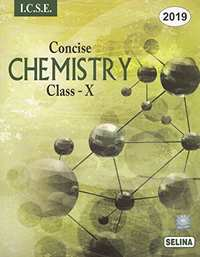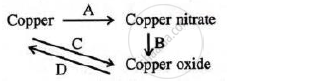Share

Selina solutions for Class 10 Chemistry chapter 10 - Study of Compounds - Nitric Acid

Selina ICSE Concise Chemistry for Class 10

Selina Selina ICSE Concise Chemistry Class 10Chapter 10: Study of Compounds - Nitric Acid

Chapter 10: Study of Compounds - Nitric Acid solutions [Page 0]

What is: (a) aqua fortis

What is: aqua regia

What is: Fixation of Nitrogen?

During thunderstorm, rain water contains nitric acid. Explain with reactions.

Write a balanced chemical equation for the laboratory preparation of nitric acid

In the preparation of nitric acid from KNO3 concentrated hydrochloric acid is not used in place of concentrated sulphuric acid. Explain why?

Conc. Nitric acid prepared in laboratory is yellow in colour why? How is this colour removed?

Give reasons for the following:
In the laboratory preparation of nitric acid, the mixture of concentrated sulphuric acid and sodium nitrate should not be heated very strongly above 200°C.

Nitric acid cannot be concentrated beyond 68% by the distillation of a dilute solution of HNO_3 State the reason.

What is passive iron? How is passivity removed?

Name the products formed when:

carbon and conc. Nitric acid is heated

Name the products formed when:

dilute HNO3 is added to copper.

Give two chemical equations for each of the following:

Reactions of nitric acid with non-metals

Give two chemical equations for each of the following:

Nitric acid showing as acidic character

Give two chemical equations for each of the following:

Nitric acid acting as oxidizing agent

Write balanced equations and name the products formed when:
Sodium hydrogen carbonate is added to nitric acid

Write balanced equations and name the products formed when:

cupric oxide reacts with nitric acid

Write balanced equations and name the products formed when:

zinc reacts with dilute nitric acid

Write balanced equations and name the products formed when:

concentrated nitric acid is heated

Write equation for the following conversions A, B, C and D.How will you prepare the following from nitric acid?

Sodium nitrate

How will you prepare the following from nitric acid?

copper nitrate

How will you prepare the following from nitric acid?

How will you prepare the following from nitric acid?

Magnesium nitrate

How will you prepare the following from nitric acid?

Ferric nitrate

How will you prepare the following from nitric acid?

Aqua regia

Correct the following, if required:

HNO_3 is strong reducing agent.

Correct the following, if required:

NaNO_3 gives Na_2 and O_2 on heating.

Correct the following, if required:

Constant boiling nitric acid contains 80% nitric acid by weight.

Correct the following, if required:

Nitric acid remains colourless even when exposed to light.

Name:

a nitrate of metal which on heating does not give nitrogen dioxide

Name:

a nitrate which on heating leaves no residue behind.

Name:

a metal nitrate which on heating is changed into metal oxide

Name:

a metal nitrate which on heating is changed into metal

Name:

a solution which absorbs nitric oxide

Name:

the oxide of nitrogen which turns brown on exposure to air. How is it prepared?

Give the chemical name and formula of the substance formed as a brown ring in the test for nitrate radical.

Mention three important uses of nitric acid. Give the property of nitric acid involved in the use.

Choose the correct answer:

The nitrate salt which does not give a mixture of NO2 and O2 on heating is:

(1) AgNO_3  (2) KNO_3
(3) Cu(NO_3)_2  (4) Zn(NO_3)_2

Choose the correct answer:

The chemical used in the brown ring test is:
(1) CuSO_4 (2) FeSO_4
(3) Fe_2(SO_4)_3 (4) N2O_5

Explain with the help of a balanced equation, the brown ring test for nitric acid.

why is freshly prepared ferrous sulphate solution used for testing the nitrate radical in the brown ring test?

From the following list of substances, choose one substance in each case which matches the description given below:

Ammonium nitrate, calcium hydrogen carbonate, copper carbonate, lead nitrate, potassium nitrate, sodium carbonate, sodium hydrogen carbonate, zinc carbonate.

A nitrate which gives off only oxygen when heated

From the following list of substances, choose one substance in each case which matches the description given below:
Ammonium nitrate, calcium hydrogen carbonate, copper carbonate, lead nitrate, potassium nitrate, sodium carbonate, sodium hydrogen carbonate, zinc carbonate.

A nitrate which on heating decomposes into dinitrogen oxide [nitrous oxide] and steam.

From the following list of substances, choose one substance in each case which matches the description given below:
Ammonium nitrate, calcium hydrogen carbonate, copper carbonate, lead nitrate, potassium nitrate, sodium carbonate, sodium hydrogen carbonate, zinc carbonate.

A nitrate which gives off oxygen and nitrogen dioxide when heated.

Fill in the blank:

Aqua regia is a mixture of 3 parts ------------ and one part ------------.

Fill in the blank:

The catalytic oxidation of ammonia to nitric oxide is ------------. (exothermic / endothermic)
process.

Fill in the blank :

Magnesium gives ------------(O2, H2, NO) with very dilute nitric acid.

Fill in the blank:

------------ (iron / copper) become passive in concentrated nitric acid

The action of heat on the blue crystalline solid A, gives a reddish brown gas B, a gas which relight a glowing splint and leaves a black residue. When gas C, which has a rotten egg smell, is passed through a solution of A, a black ppt. is formed.
(a) Identify A, B and C
(b) Write equation for action of heat on A.
(c) Write equation between solution of A and gas C.

The action of heat on the blue crystalline solid A, gives a reddish brown gas B, a gas which relight a glowing splint and leaves a black residue. When gas C, which has a rotten egg smell, is passed through a solution of A, a black ppt. is formed.
(a) Identify A, B and C
(b) Write equation for action of heat on A.
(c) Write equation between solution of A and gas C.

Chapter 10: Study of Compounds - Nitric Acid

Selina Selina ICSE Concise Chemistry Class 10Selina solutions for Class 10 Chemistry chapter 10 - Study of Compounds - Nitric Acid

Selina solutions for Class 10 Chemistry chapter 10 (Study of Compounds - Nitric Acid) include all questions with solution and detail explanation. This will clear students doubts about any question and improve application skills while preparing for board exams. The detailed, step-by-step solutions will help you understand the concepts better and clear your confusions, if any. Shaalaa.com has the CISCE Selina ICSE Concise Chemistry for Class 10 solutions in a manner that help students grasp basic concepts better and faster.

Further, we at Shaalaa.com are providing such solutions so that students can prepare for written exams. Selina textbook solutions can be a core help for self-study and acts as a perfect self-help guidance for students.

Concepts covered in Class 10 Chemistry chapter 10 Study of Compounds - Nitric Acid are Nitric Acid as an Oxidizing Agent., Nitric Acid - Laboratory Method of Preparation of Nitric Acid from Potassium Nitrate Or Sodium Nitrate, Concept of Nitric Acid.

Using Selina Class 10 solutions Study of Compounds - Nitric Acid exercise by students are an easy way to prepare for the exams, as they involve solutions arranged chapter-wise also page wise. The questions involved in Selina Solutions are important questions that can be asked in the final exam. Maximum students of CISCE Class 10 prefer Selina Textbook Solutions to score more in exam.

Get the free view of chapter 10 Study of Compounds - Nitric Acid Class 10 extra questions for Chemistry and can use Shaalaa.com to keep it handy for your exam preparation

S